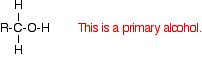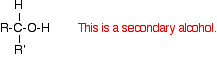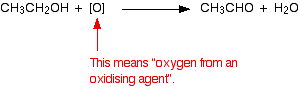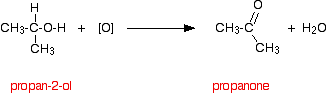Need help? Email help@shout.education

# The Preparation of Aldehydes and Ketones

This page explains how aldehydes and ketones are made in the lab by the oxidation of primary and secondary alcohols.

## Oxidising Alcohols to Make Aldehydes and Ketones

### General

The oxidising agent used in these reactions is normally a solution of sodium or potassium dichromate(VI) acidified with dilute sulfuric acid. If oxidation occurs, the orange solution containing the dichromate(VI) ions is reduced to a green solution containing chromium(III) ions.

The net effect is that an oxygen atom from the oxidising agent removes a hydrogen from the -OH group of the alcohol and one from the carbon to which it is attached.[O] is often used to represent oxygen coming from an oxidising agent.

R and R' are alkyl groups or hydrogen. They could also be groups containing a benzene ring, but I'm ignoring these to keep things simple.

If at least one of these groups is a hydrogen atom, then you will get an aldehyde. If they are both alkyl groups then you get a ketone.

If you now think about where they are coming from, you will get an aldehyde if your starting molecule looks like this:In other words, if you start from a primary alcohol, you will get an aldehyde.

You will get a ketone if your starting molecule looks like this:where R and R' are both alkyl groups.

Secondary alcohols oxidise to give ketones.

### Making Aldehydes

Aldehydes are made by oxidising primary alcohols. There is, however, a problem.

The aldehyde produced can be oxidised further to a carboxylic acid by the acidified potassium dichromate(VI) solution used as the oxidising agent. In order to stop at the aldehyde, you have to prevent this from happening.

Note: This further oxidation is explained in more detail on the page about oxidation of alcohols.

To stop the oxidation at the aldehyde, you

• use an excess of the alcohol. That means that there isn't enough oxidising agent present to carry out the second stage and oxidise the aldehyde formed to a carboxylic acid.
• distil off the aldehyde as soon as it forms. Removing the aldehyde as soon as it is formed means that it doesn't stay in the mixture to be oxidised further.

If you used ethanol as a typical primary alcohol, you would produce the aldehyde ethanal, CH3CHO.

The full equation for this reaction is fairly complicated, and you need to understand about electron-half-equations in order to work it out.

3\text{CH}_3\text{CH}_2\text{OH} + {\text{Cr}_2\text{O}_7}^{2-} + 8\text{H}^+ \longrightarrow 3\text{CH}_3\text{CHO} + 2\text{Cr}^{3+} + 7\text{H}_2\text{O}

Note: If you should know about electron-half-equations but aren't happy about them, you could follow this link. This particular equation isn't worked out on that page, but there should be enough information there to enable you to do it if you wanted to.

In organic chemistry, simplified versions are often used which concentrate on what is happening to the organic substances. To do that, oxygen from an oxidising agent is represented as [O]. That would produce the much simpler equation:

\text{CH}_3\text{CH}_2\text{OH} + \underbrace{[\text{O}]}_{\clap{\color{#467abf}{\text{the means "oxygen from an oxidising agent"}}}{}} \longrightarrow \text{CH}_3\text{CH}_2\text{H}_2\text{O}### Secondary Alcohols

Secondary alcohols are oxidised to ketones. There is no further reaction which might complicate things. For example, if you heat the secondary alcohol propan-2-ol with sodium or potassium dichromate(VI) solution acidified with dilute sulfuric acid, you get propanone formed.

Playing around with the reaction conditions makes no difference whatsoever to the product.

Using the simple version of the equation: